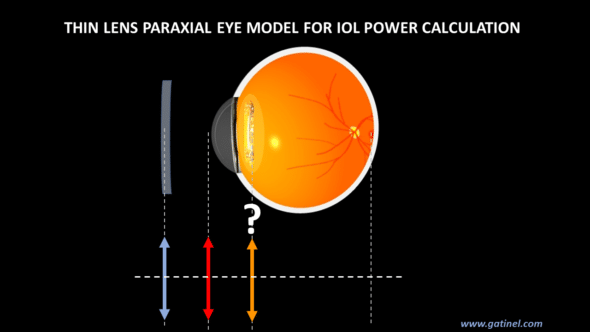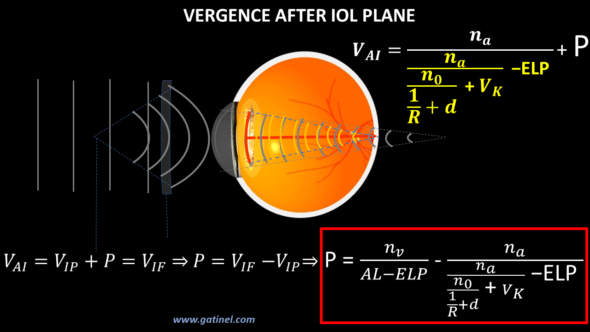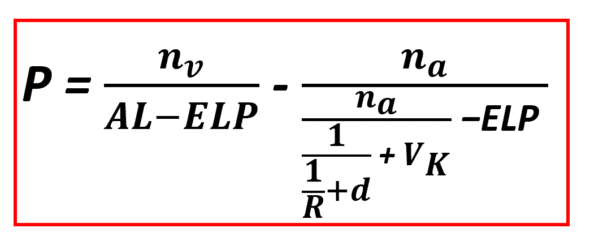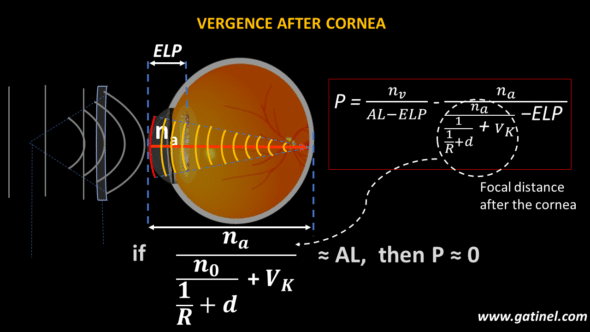+ +

# 4. Thin lens IOL power computation

This page is devoted to developing a theoretical formula for calculating an intraocular lens (IOL) power from a paraxial eye model made up of two thin lenses (the cornea and the IOL whose power we want to calculate to achieve the desired postoperative refraction). This formula is the core of many IOL power calculation formulas.

It is possible to aim for non-zero postoperative refraction, and in this case, the pseudophakic eye sees clearly in the distance through a corrective spectacle lens.To establish the thin lens IOL power formula, the reader is expected to know the definition of vergence and be relatively familiar with calculating vergence step-by-step as the light emitted from a distant source propagates through the ocular media. In short, when a wavefront meets a refractive element (cornea or IOL), we add the value of the vergence of this element under the vergence formula. When the wavefront propagates in a medium, we subtract or add a segment to the distance to the focus, which corresponds to the distance traveled by the wavefront in the medium.

As we will see later, the formula for calculating the power of an implant is equal to the subtraction of vergences.

## Vergence after refraction by the IOL in an emmetropic or well-corrected eye

The vergence at a given location is equal to the ratio between the refractive index of the propagation medium and the distance to the focus. Thus, in the case of an emmetropic eye or one corrected for its refractive error in glasses, the vergence of a light wavefront emitted by a point source after successive refractions by the cornea and the  IOL must be centered on the fovea.

Just after passing the IOL, the vergence VIF of an emmetropic or well-corrected pseudophakic eye must be equal to the ratio between the vitreous refractive index and the distance to the fovea from the IOL. This distance is equal to the difference between the axial length and the distance between the cornea and the IOL, none other than the effective lens position (ELP).

To obtain an expression making it possible to calculate the power of the IOL intended to obtain the desired postoperative refraction, we will calculate the value of the vergence propagated from a source point located at infinity to the intraocular lens (more precisely at the output of it). Once this expression is established, we will then set equality with the VIF vergence established above and solve it for the vergence of the IOL.

## Vergence of the incoming wavetrain

Let’s start by establishing the value of the vergence of a wave train coming from the distant source in contact with a spectacle lens, correcting our pseudophakic eye. Due to the considerable distance between the source and the spectacle lens, this vergence is zero. The incident wavefront is plane.

## Vergence after refraction by the spectacle glass

The power of this spectacle lens is equal (in absolute value) to the target postoperative refraction R (which is generally, in the context of a desirable post-surgical refractive outcome between emmetropia –> Plano lens = 0D, and low myopia for reading –> -2.50 D). As soon as the wavefront emerges from the spectacle lens, its vergence is equal to this spectacle lens power.

This vergence corresponds to the inverse of the distance from/to the focus. In the case of a concave glass (correcting myopia), the focus is on the left, the wavefront is diverging, and the vergence is negative.

## Vergence at the corneal plane

At the corneal plane, the vergence (VCP) is easily calculated as the inverse of the sum of the distance to the focus (fs) with the distance between the spectacle lens and the cornea (d). This vergence has a negative sign (divergent incoming light).

## Vergence after refraction by the cornea

After the cornea, the vergence VAC is obtained by adding to the vergence at the corneal plane the vergence of the cornea. A change in the refractive index takes place since light travels in the aqueous humor. The distance to the new focus is obtained by dividing the refractive index of the aqueous humor (na) by the vergence after the cornea (VAC). It is positive (converging to the right).

## Vergence at IOL plane

It is now necessary to calculate the vergence of the wavefront reaching the plane of the IOL (VIP: vergence at IOL plane). It suffices to subtract the distance corresponding to the space between the cornea and the IOL from the previously calculated focusing distance (na/VAC). This space, unknown before the surgery, is the “Effective Lens Position”(ELP). For numerical applications, such as calculating the IOL’s power, which interests us here, one must choose a predicted value for the ELP since it can only be anticipated.

Different methods make it possible to increase the quality of the ELP prediction. They all have one thing in common; they are based on the retrospective study of well-documented case series of pseudophakic eyes. If we know the preoperative biometric characteristics, the power, and the refractive outcome for a given eye, we can back-calculate the value of the ELP. We can include such information in a large database, making it possible to apply regression methods or develop machine learning algorithms.

In a thin lens eye model, the ELP corresponds to the anticipated distance between the corneal and IOL planes. On the other hand, if the thickness of the cornea and/or the implant is taken into account, this distance must be revised according to the rules established for thick lens paraxial models.

## Vergence after refraction by the IOL

Our last step is to calculate the vergence after refraction by the IOL (VAI). Under the vergence formula, it suffices to add the IOL’s power to the vergence calculated previously (VAI, the vergence at IOL plane). The expression obtained is equal to VIF if the starting condition is verified: the IOL power (P) provides the eye with the desired refraction R.Solving the equation for the value P provides an IOL power calculation formula intended to induce the refraction R (R=0 when targeting emmetropia) in the air (n0=1) for an eye of axial length AL and total corneal power VK.Again, one must choose a value for the ELP in advance: the modalities and determinants of this choice constitute what still differentiates most of the theoretical implant calculation formulas today.

As underlined in the introduction, the formula that provides the implant’s power is a subtraction of vergences, as evidenced by the presence of a refractive index value in the numerator and a distance in the denominator of the fractions.

The structure of the equation is pretty straightforward. The first term is the vergence after the implant (VIF), the second is the vergence after the cornea propagated in the plane of the expected position of the implant (ELP). Therefore, if these two vergences are equal, the power of the IOL is zero. This logically occurs when the distance to the focus of a wavefront refracted by the cornea is equal to the axial length of the eye considered.Consider a value for VK equal to 43D. The theoretical distance to the focal point after the cornea is equal to 1.337 / 43 ≈ 31 mm: it is effectively a value close to what we anticipate for the axial length of an eye with high myopia, for which an IOL power close to 0 would be required for reach emmetropia (R=0).

The aim is often to induce slight postoperative myopia (e.g., R = -2.50D). In this hypothesis, the theoretical value of the axial length of an eye provided with a cornea of vergence VK = 40.50D for which an implant of zero power would induce a refraction R = -2.50D is equal to 1.337 / 40.5 ≈ 33 mm.

The spreadsheet below allows you to calculate the theoretical value of the IOL power for the preoperative biometric values and an anticipated value of the ELP.

## Use the following spreadsheet to compute the IOL power using this established formula:

This page allowed us to establish and use an IOL power calculation formula using the vergence formula on a paraxial eye model with thin lenses. The IOLs are certainly provided with a small thickness, but this is not negligible because of the order of mm. To gain precision, it is interesting to consider an eye model with thick lenses for the next steps. However, this requires familiarization with certain notions, such as the principal plane, which will be the subject of our next attention.

### 2 réponses à “4. Thin lens IOL power computation”

1.Dr Damien Gatinel dit :

A thin lens is a simplified theoretical system that has no curvature or refractive index and zero thickness. Of course, a « real » lens is made of a material that has a certain index and curvatures. In this case, one can apply the paraxial formulas specific to thick lenses to calculate the object and image focal lengths (these formulas are reported here: https://www.gatinel.com/paraxial-power-of-the-iol/ within the framework of the study of the paraxial properties of the intraocular implants). Once these distances are known, the thick lens can be replaced by a thin lens whose vergence is calculated using the position of the foci (the inverse of this distance if the lens is in the air).

2.Nahed dit :

Thank you for the valuable information.
Could you please send me the definition of vergence of a thin lens in terms of its refractive index and radius of curvature? Assuming equal radii and placement in air, does this relation lead to the known formula states the the focal length equals 0.5 the radius of curvature?

Warning: error_log(/home/clients/0e98f30bad73cbe357ff222deca9aba0/web/gatinel/wp-content/mc_data/53c4b42756475b28ee65f2b1a786a741-mc.log): failed to open stream: Disk quota exceeded in /home/clients/0e98f30bad73cbe357ff222deca9aba0/web/gatinel/wp-content/plugins/wpremote/protect/logger/fs.php on line 16